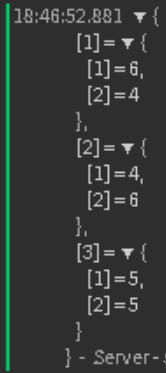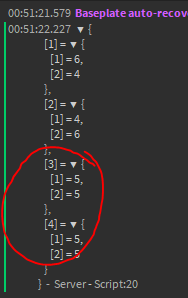# How do you find all pairs of an integer array whose sum is equal to a given number?

Hey guys,

In my opinion this is hard to solve, anyways for you not?

What I want to do?

How do you find all pairs of an integer array whose sum is equal to a given number.

For example, if the input integer array is {2, 7, 3, 9, 11} and given sum is 10, the output should be {7,3}.

So you may think this looks easy to solve but dont think about it, if you think long then you know that this problem sounds not easy - when you never code something like that.
This is what I have been created so far but it doesnt seem work well, maybe you find the problem or the mistake I did here.

``````local numbers = {3,6,4,2,1,5,2}

function sumwewant(array, sum)
for i = 1, #array do
local first = array[i]
for j = 1, #array do
local second = numbers[j]
if ((first + second) == sum)then
print(first, second)
end
end
end
end

local d  = sumwewant(numbers, 10)
print(d)
``````
1 Like

This is an interesting and hard question to answer. This is the best I can do.

``````local function(array,sumWeWant)
for i = 1, #array, do
local first = array
for j = (i + 1), #array do
local second = array(j)
local result = first + second
if result == sumWeWant then
return result
else return end
end
end
end
``````

I think this is what you’re looking for.

1 Like

Output is:

ServerScriptService.Script:7: attempt to call a table value

``````local numbers = {3,6,4,2,1,5,2}

local function findarray(array,sumWeWant)
for i = 1, #array do
local first = array
for j = (i + 1), #array do
local second = array(j)
local result = first + second
local d = sumWeWant
if result == result then
return result
else return end
end
end
end

local d = findarray(numbers, 10)
print(d)
``````
1 Like

Whoops, not suppose to be brackets AKA (), should be square ones AKA [].

1 Like

Whoops, not suppose to be brackets AKA (), should be square ones AKA [].

I cant follow you AKA? () → [] I am a bit lost

1 Like

Just change

``````array(j)
``````

To

``array[j]``
1 Like

I don’t see the issue, your code works fine

1 Like

oh yes it must be [], but anyways.
still got nill

``````local numbers = {3,6,4,2,1,5,2}

local function findarray(array,sumWeWant)
for i = 1, #array do
local first = array
for j = (i + 1), #array do
local second = array[j]
local result = first + second
local de = sumWeWant
if result == de then
return result
else return end
end
end
end

local d = findarray(numbers, 10)
print(d)
``````
1 Like

Are you trying to make “findarray” return an array of all the number pairs?

which one you mean my or ItzMezeus?

Your code works fine. If the issue is that it’s printing `nil` then change the function to return an array with all the matched number because your function isn’t returning anything

``````local numbers = {3,6,4,2,1,5,2}

function sumwewant(array, sum)
local matches = {}
for i = 1, #array do
local first = array[i]
for j = 1, #array do
local second = numbers[j]
if ((first + second) == sum)then
table.insert(matches, {first, second})
end
end
end
return matches
end

local d  = sumwewant(numbers, 10)
print(d)
``````but where comes the 5, 5 in the table is nowhere a 5, 5
I mean if you look at my array

That’s because it’s checking the same number twice, in the 2nd loop you should add a check so i ~= j

``````local numbers = {3,6,4,2,1,5,2}

function sumwewant(array, sum)
local matches = {}
for i = 1, #array do
local first = array[i]
for j = 1, #array do
if i ~= j then
local second = numbers[j]
if ((first + second) == sum)then
table.insert(matches, {first, second})
end
end
end
end
return matches
end

local d  = sumwewant(numbers, 10)
print(d)
``````I knew it loop different but actually I cant figure out how to make it work well for loop

this isnt good what if I want add in my table a 5 now?

yea I think you know what would happenYou could check if the pair already exists and skip over it